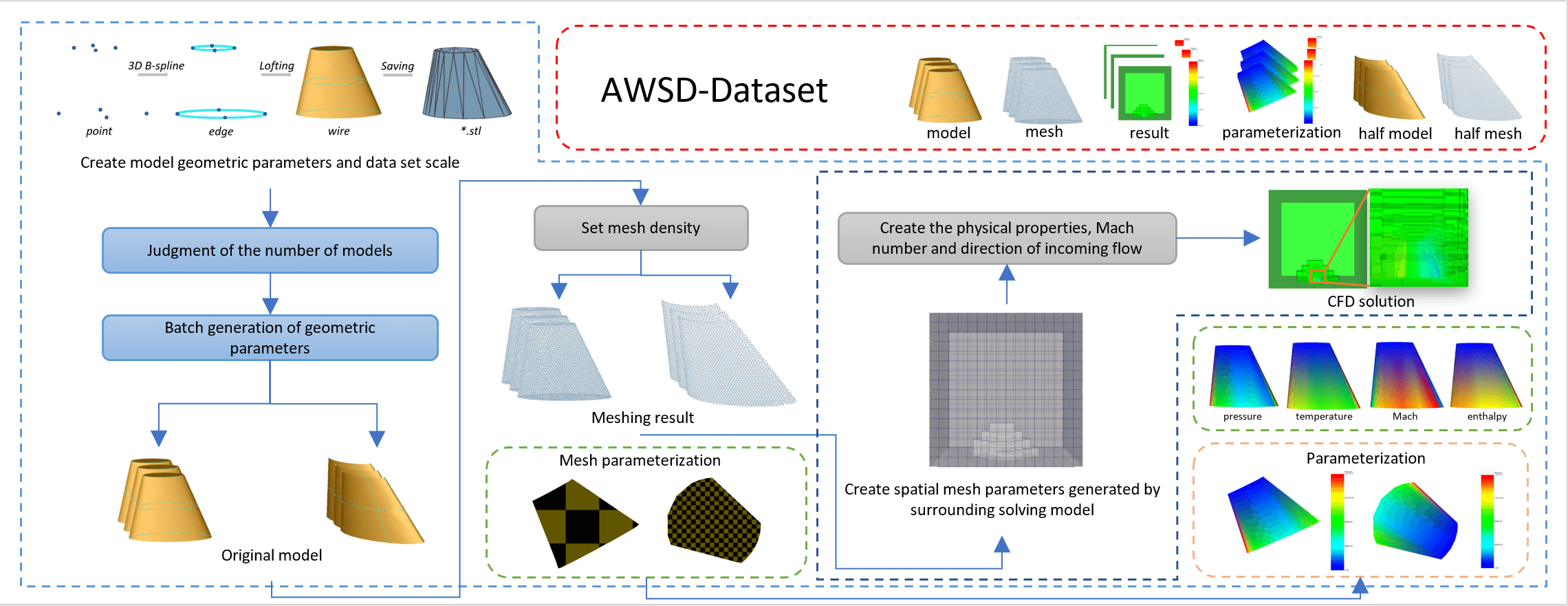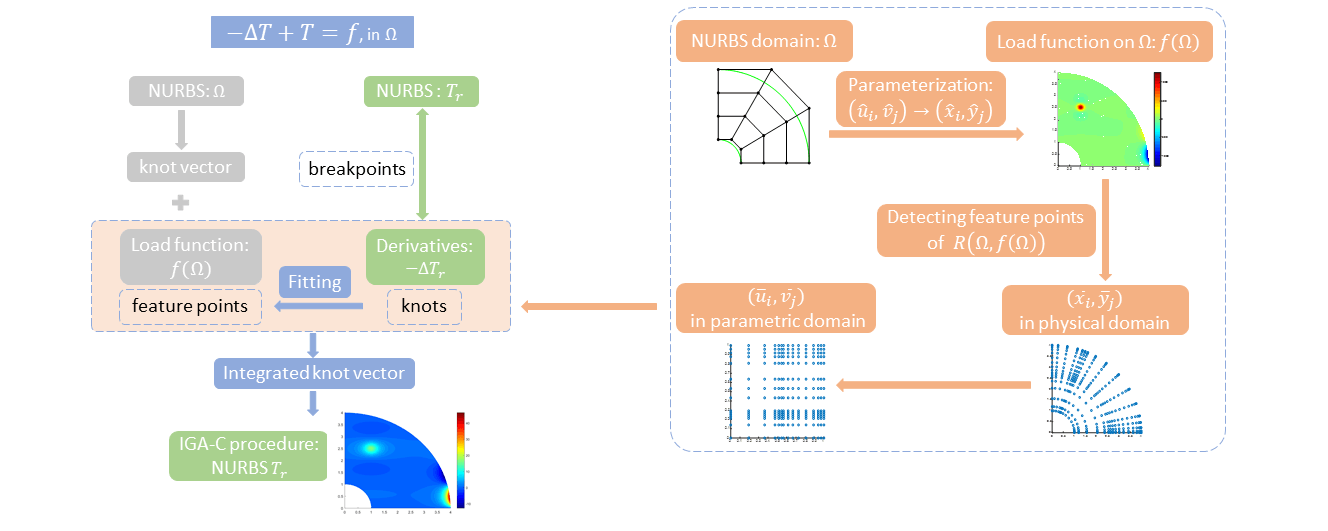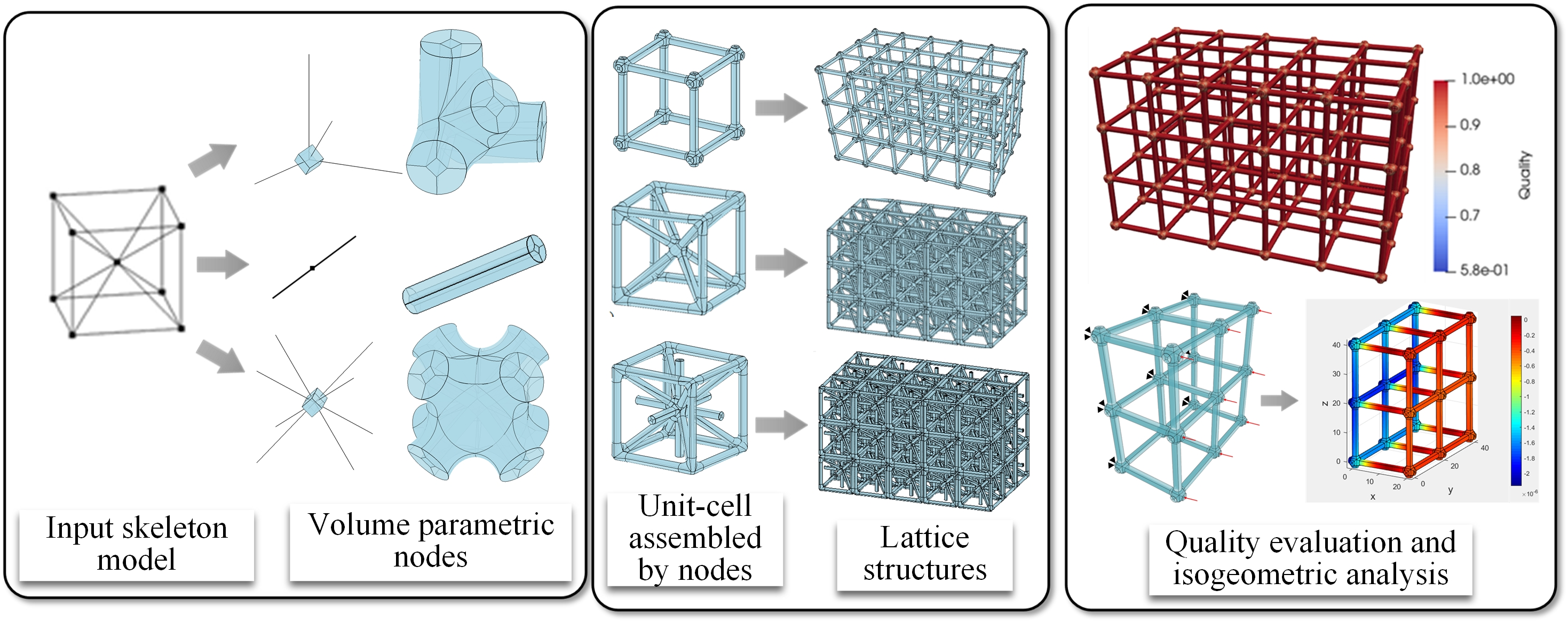Special lssues
Table of Content

# Integration of Geometric Modeling and Numerical Simulation

Submission Deadline: 31 December 2022 (closed)

### Guest Editors

Prof. Gang Xu, Hangzhou Dianzi University, China
Prof. Stéphane P. A Bordas, University of Luxembourg, Luxembourg
Prof. Xiaodong Wei, Shanghai Jiao Tong University, China

### Summary

In the past decade, the integration of Geometric Modeling technology and Numerical Simulation method, such as the iso-geometric analysis and its variants, has been an active area of research, due to their advantages in many aspects such as high-order accuracy, consistent geometric data representation as well as efficient refinement operation. This integration not only provides powerful numerical simulation approaches for industrial applications, but also opens new research direction in the field of geometric modeling and computer graphics. This special issue focuses on the integration of geometric modeling methods and numerical analysis theory, while also addressing the application of this integration. In addition to the strong focus in isogeometric analysis and its engineering application, a special emphasis will be given to geometric modeling and mesh generation issues for numerical simulation.

Potential topics include, but are not limited to:

• Spline curve and surface modeling

• Trivariate volumetric modeling

• Subdivision surface/volumes

• Mesh generation for simulation

• High-order mesh generation

• Volumetric parameterization

• Analysis-suitable parameterization

• Analysis-suitable spline spaces

• Generalized iso-geometric analysis methods

• Refinement methods in iso-geometric analysis

• Numerical analysis of iso-geometric methods

• Fast iso-geometric solver

• Engineering application with iso-geometric analysis

• High-order finite element method for PDEs

• Meshless methods in computational mechanics

• Deep-learning methods in computational mechanics

• Iso-geometric boundary element methods

• Iso-geometric shape optimization methods

• Iso-geometric topology optimization methods

### Keywords

Geometric modeling; mesh generation, numerical simulation; isogeometric analysis; shape optimization; topology optimization

### Published Papers

•Open Access

ARTICLE

New Soliton Wave Solutions to a Nonlinear Equation Arising in Plasma Physics

CMES-Computer Modeling in Engineering & Sciences, DOI:10.32604/cmes.2023.027344
（This article belongs to this Special Issue: Integration of Geometric Modeling and Numerical Simulation)
Abstract The extraction of traveling wave solutions for nonlinear evolution equations is a challenge in various mathematics, physics, and engineering disciplines. This article intends to analyze several traveling wave solutions for the modified regularized long-wave (MRLW) equation using several approaches, namely, the generalized algebraic method, the Jacobian elliptic functions technique, and the improved Q-expansion strategy. We successfully obtain analytical solutions consisting of rational, trigonometric, and hyperbolic structures. The adaptive moving mesh technique is applied to approximate the numerical solution of the proposed equation. The adaptive moving mesh method evenly distributes the points on the high error areas. This method perfectly and… More >

•Open Access

ARTICLE

AWSD: An Aircraft Wing Dataset Created by an Automatic Workflow for Data Mining in Geometric Processing

CMES-Computer Modeling in Engineering & Sciences, Vol.136, No.3, pp. 2935-2956, 2023, DOI:10.32604/cmes.2023.026083
（This article belongs to this Special Issue: Integration of Geometric Modeling and Numerical Simulation)
Abstract This paper introduces an aircraft wing simulation data set (AWSD) created by an automatic workflow based on creating models, meshing, simulating the wing flight flow field solution, and parameterizing solution results. AWSD is a flexible, independent wing collection of simulations with specific engineering requirements. The data set is applicable to handle computer geometry processing tasks. In contrast to the existing 3D model data set, there are some advantages the scale of this data set is not limited by the collection source, the data files have high quality, no defects, redundancy, and other problems, and the models and simulation are all… More >

Graphic Abstract•Open Access

ARTICLE

New Perspective to Isogeometric Analysis: Solving Isogeometric Analysis Problem by Fitting Load Function

CMES-Computer Modeling in Engineering & Sciences, Vol.136, No.3, pp. 2957-2984, 2023, DOI:10.32604/cmes.2023.025983
（This article belongs to this Special Issue: Integration of Geometric Modeling and Numerical Simulation)
Abstract Isogeometric analysis (IGA) is introduced to establish the direct link between computer-aided design and analysis. It is commonly implemented by Galerkin formulations (isogeometric Galerkin, IGA-G) through the use of nonuniform rational B-splines (NURBS) basis functions for geometric design and analysis. Another promising approach, isogeometric collocation (IGA-C), working directly with the strong form of the partial differential equation (PDE) over the physical domain defined by NURBS geometry, calculates the derivatives of the numerical solution at the chosen collocation points. In a typical IGA, the knot vector of the NURBS numerical solution is only determined by the physical domain. A new perspective… More >

Graphic Abstract•Open Access

ARTICLE

Automatic Extraction of the Sparse Prior Correspondences for Non-Rigid Point Cloud Registration

CMES-Computer Modeling in Engineering & Sciences, Vol.136, No.2, pp. 1835-1856, 2023, DOI:10.32604/cmes.2023.025662
（This article belongs to this Special Issue: Integration of Geometric Modeling and Numerical Simulation)
Abstract Non-rigid registration of point clouds is still far from stable, especially for the largely deformed one. Sparse initial correspondences are often adopted to facilitate the process. However, there are few studies on how to build them automatically. Therefore, in this paper, we propose a robust method to compute such priors automatically, where a global and local combined strategy is adopted. These priors in different degrees of deformation are obtained by the locally geometrical-consistent point matches from the globally structural-consistent region correspondences. To further utilize the matches, this paper also proposes a novel registration method based on the Coherent Point Drift… More >

•Open Access

ARTICLE

Isogeometric Analysis of Longitudinal Displacement of a Simplified Tunnel Model Based on Elastic Foundation Beam

CMES-Computer Modeling in Engineering & Sciences, Vol.136, No.1, pp. 803-824, 2023, DOI:10.32604/cmes.2023.024833
（This article belongs to this Special Issue: Integration of Geometric Modeling and Numerical Simulation)
Abstract Serious uneven settlement of the tunnel may directly cause safety problems. At this stage, the deformation of the tunnel is predicted and analyzed mainly by numerical simulation, while the commonly used finite element method (FEM) uses low-order continuous elements. Therefore, the accuracy of tunnel settlement prediction is not enough. In this paper, a method is proposed to study the vertical deformation of the tunnel by using the combination of isogeometric analysis (IGA) and Bézier extraction operator. Compared with the traditional IGA method, this method can be easily integrated into the existing FEM framework, and ensure the same accuracy. A numerical… More >

•Open Access

ARTICLE

A Geometrically Exact Triangular Shell Element Based on Reproducing Kernel DMS-Splines

CMES-Computer Modeling in Engineering & Sciences, Vol.136, No.1, pp. 825-860, 2023, DOI:10.32604/cmes.2023.022774
（This article belongs to this Special Issue: Integration of Geometric Modeling and Numerical Simulation)
Abstract To model a multibody system composed of shell components, a geometrically exact Kirchhoff-Love triangular shell element is proposed. The middle surface of the shell element is described by using the DMS-splines, which can exactly represent arbitrary topology piecewise polynomial triangular surfaces. The proposed shell element employs only nodal displacement and can automatically maintain C1 continuity properties at the element boundaries. A reproducing DMS-spline kernel skill is also introduced to improve computation stability and accuracy. The proposed triangular shell element based on reproducing kernel DMS-splines can achieve an almost optimal convergent rate. Finally, the proposed shell element is validated via three… More >

Graphic Abstract•Open Access

ARTICLE

Skeleton-Based Volumetric Parameterizations for Lattice Structures

CMES-Computer Modeling in Engineering & Sciences, Vol.135, No.1, pp. 687-709, 2023, DOI:10.32604/cmes.2022.021986
（This article belongs to this Special Issue: Integration of Geometric Modeling and Numerical Simulation)
Abstract Lattice structures with excellent physical properties have attracted great research interest. In this paper, a novel volume parametric modeling method based on the skeleton model is proposed for the construction of three-dimensional lattice structures. The skeleton model is divided into three types of nodes. And the corresponding algorithms are utilized to construct diverse types of volume parametric nodes. The unit-cell is assembled with distinct nodes according to the geometric features. The final lattice structure is created by the periodic arrangement of unit-cells. Several different types of volume parametric lattice structures are constructed to prove the stability and applicability of the… More >

Graphic Abstract